Math Olympiad Test: Number System- 1

# Math Olympiad Test: Number System- 1

Test Description

## 10 Questions MCQ Test Mathematics Olympiad for Class 9 | Math Olympiad Test: Number System- 1

Math Olympiad Test: Number System- 1 for Class 9 2022 is part of Mathematics Olympiad for Class 9 preparation. The Math Olympiad Test: Number System- 1 questions and answers have been prepared according to the Class 9 exam syllabus.The Math Olympiad Test: Number System- 1 MCQs are made for Class 9 2022 Exam. Find important definitions, questions, notes, meanings, examples, exercises, MCQs and online tests for Math Olympiad Test: Number System- 1 below.
Solutions of Math Olympiad Test: Number System- 1 questions in English are available as part of our Mathematics Olympiad for Class 9 for Class 9 & Math Olympiad Test: Number System- 1 solutions in Hindi for Mathematics Olympiad for Class 9 course. Download more important topics, notes, lectures and mock test series for Class 9 Exam by signing up for free. Attempt Math Olympiad Test: Number System- 1 | 10 questions in 10 minutes | Mock test for Class 9 preparation | Free important questions MCQ to study Mathematics Olympiad for Class 9 for Class 9 Exam | Download free PDF with solutions
 1 Crore+ students have signed up on EduRev. Have you?
Math Olympiad Test: Number System- 1 - Question 1

### Choose the correct statement:

Detailed Solution for Math Olympiad Test: Number System- 1 - Question 1

Zero is a whole number which is not a natural number. Every integer is a rational number. Every whole number is an integer but converse is false.

Math Olympiad Test: Number System- 1 - Question 2

### Which of the following decimal is terminating?

Detailed Solution for Math Olympiad Test: Number System- 1 - Question 2

∵ All the fractions are in their simplest form.
∴ The fraction having the denominator in the form 2m × 5n will be terminating.
∴ Just analyzing the denominators, we have 11, 6, and 7 cannot be expressed in 2m × 5n form, but 16 = 24 × 50.
∴ 11/16 will be a terminating decimal.

Math Olympiad Test: Number System- 1 - Question 3

### Which of the following number is rational?

Detailed Solution for Math Olympiad Test: Number System- 1 - Question 3

π = 3.14157...... (Non-repeating non-terminating decimal)
22/7 = 3.142871
∴ 22/7 is a rational number.

Math Olympiad Test: Number System- 1 - Question 4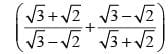× 50 equals

Detailed Solution for Math Olympiad Test: Number System- 1 - Question 4

Here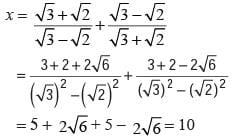∴ Required value = 10 × 50 = 500

Math Olympiad Test: Number System- 1 - Question 5

The value of the expression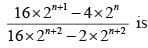Detailed Solution for Math Olympiad Test: Number System- 1 - Question 5

We have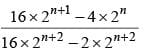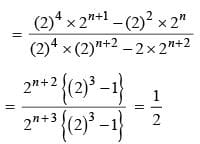Math Olympiad Test: Number System- 1 - Question 6

If 5x-3 ⋅ 32x-8 = 225, x = ?

Detailed Solution for Math Olympiad Test: Number System- 1 - Question 6

Given 5x-3 ⋅ 32x-8 = 225 = 52 . 32
∴ x – 3 = 2x – 8 = 2
⇒ x = 5

Math Olympiad Test: Number System- 1 - Question 7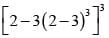= x then the value of x = ?

Detailed Solution for Math Olympiad Test: Number System- 1 - Question 7

Here [ 2 - 3( 2 - 3)3]3 = [ 2 - 3(-1)3]3
= [ 2 + 3]3 = (5)3 = 125 = x

Math Olympiad Test: Number System- 1 - Question 8

If x2 +  1/x2 = 98, then  x + 1/x = ?

Detailed Solution for Math Olympiad Test: Number System- 1 - Question 8

Here ( x + 1/x)2 = x2 + 1/x2 + 2
= (98 + 2) =100
⇒  x + 1 = √100 = 10

Math Olympiad Test: Number System- 1 - Question 9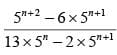is equal to

Detailed Solution for Math Olympiad Test: Number System- 1 - Question 9

Here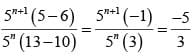Math Olympiad Test: Number System- 1 - Question 10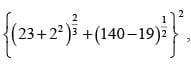is

Detailed Solution for Math Olympiad Test: Number System- 1 - Question 10

The given equation can be written as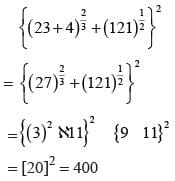## Mathematics Olympiad for Class 9

1 videos|43 tests
 Use Code STAYHOME200 and get INR 200 additional OFF Use Coupon Code
Information about Math Olympiad Test: Number System- 1 Page
In this test you can find the Exam questions for Math Olympiad Test: Number System- 1 solved & explained in the simplest way possible. Besides giving Questions and answers for Math Olympiad Test: Number System- 1, EduRev gives you an ample number of Online tests for practice

## Mathematics Olympiad for Class 9

1 videos|43 tests